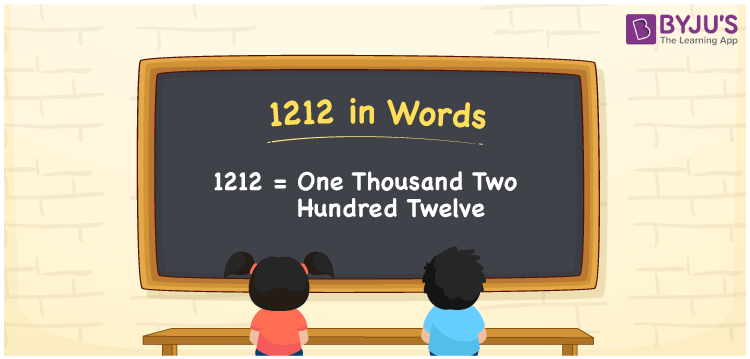# 1212 in Words

We can write 1212 in words as One Thousand Two Hundred Twelve. Suppose you bought a new specs that costs Rs. 1212, then you could say that “ I bought new specs for One Thousand Two Hundred Twelve rupees”. In this article, you will learn how to convert the number 1212 into words in an exciting way. The number 1212 is used in expressions that relate to money, days, distance, length, weight and so on.

 1212 in words One Thousand Two Hundred Twelve One Thousand Two Hundred Twelve in Numbers 1212

## 1212 in English Words

We generally write numbers in words using the English alphabet. Thus, we can read 1212 in English as “One Thousand Two Hundred Twelve”.## How to Write 1212 in Words?

In this section, you will learn how to write the number 1212 in words. The given number 1212 has four digits, so we should make a place value chart that represents the place value for all these four digits. The order of place values of digits in a number is in accordance with the Indian numbering system, such as:

• Ones
• Tens
• Hundreds
• Thousands
• Ten-thousands
• Hundred-thousands or Lakhs

This can be done as follows.

 Thousands Hundreds Tens Ones 1 2 1 2

Thus, we can write the expanded form as:

1 × Thousand + 2 × Hundred + 1 × Ten + 2 × One

= 1 × 1000 + 2 × 100 + 1 × 10 + 2 × 1

= 1212

= One thousand five hundred

1212 is a natural number that precedes 1213 and succeeds 1211.

1212 in words – One Thousand Two Hundred Twelve

Is 1212 an odd number? – No

Is 1212 an even number? – Yes

Is 1212 a perfect square number? – No

Is 1212 a perfect cube number? – No

Is 1212 a prime number? – No

Is 1212 a composite number? – Yes

## Frequently Asked Questions on 1212 in Words

Q1

### How do you write 1212 in English?

We can write 1212 in English words as “One Thousand Two Hundred Twelve”.
Q2

### How do you write 1212 in words for a cheque?

For a cheque, we generally write 1212 in words as One Thousand Two Hundred Twelve rupees only.
Q3

### What number is 1212 in words?

The number 1212 is One Thousand Two Hundred Twelve in words.